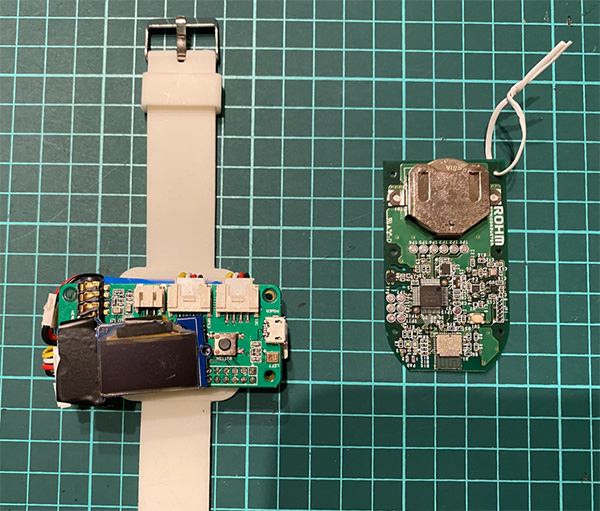## AE-BME280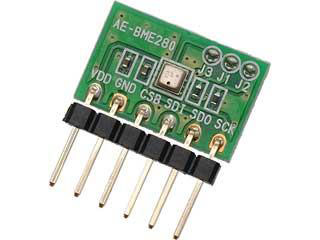BME280——一块位于元件中心的微小银芯片——承担了大部分工作。该模块表面上还有一个非常小的开口，其作用是读取数值，因此切勿遮盖这个开口。

AE-BME280板和排针本来就是分开的。连接Raspberry Pi的最简单方法就是将两者组装在一起，如图1所示。这需要焊接。我购买的排针有10个引脚，但是连接AE-BME280只需6个引脚，所以应剪掉第6个引脚后面的排针。

I2C – 维基百科
I2C（集成电路总线）是一种由Philips Semiconductor（现为NXP Semiconductors）发明的串行总线。I2C代表I-平方-C。由于纯文本环境中的字符限制，我们将其称为I2C或IIC。该协议通常用于将低速外围IC连至主板、嵌入式系统和移动电话等设备。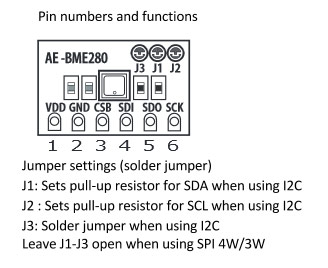## 焊接!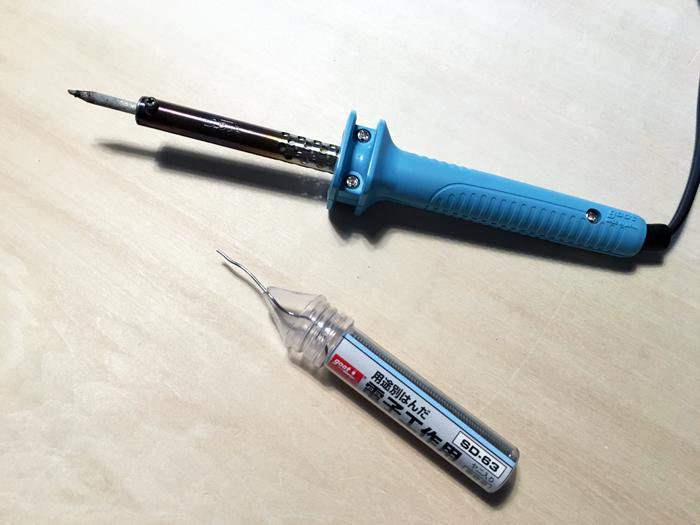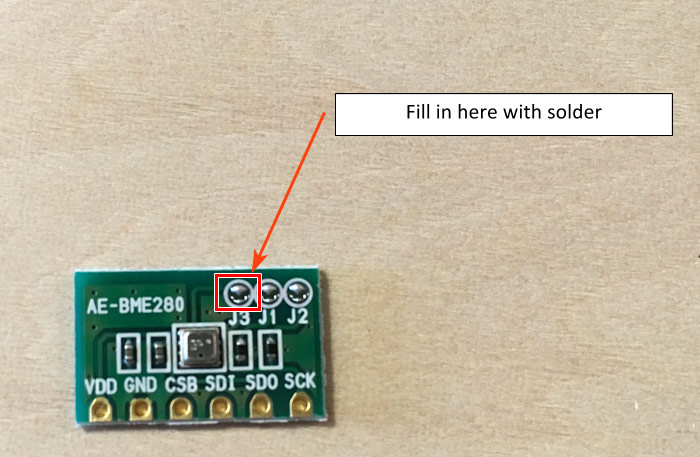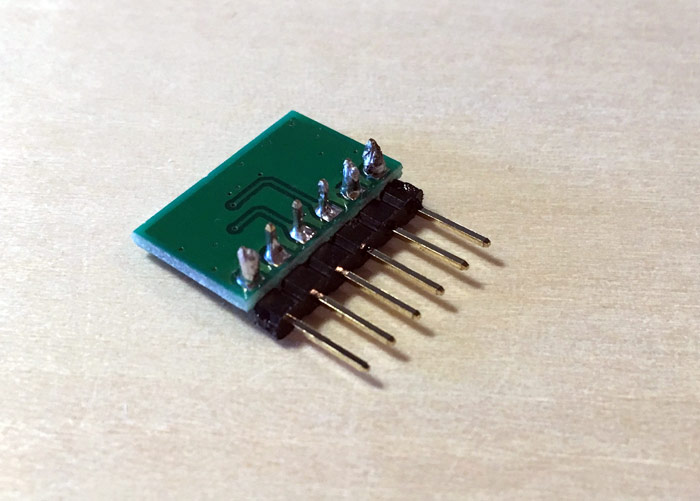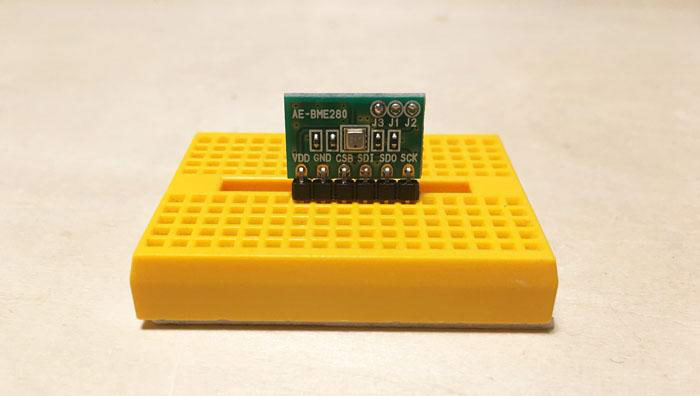## 接线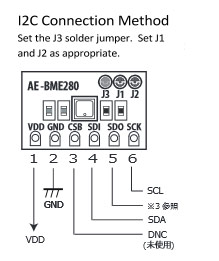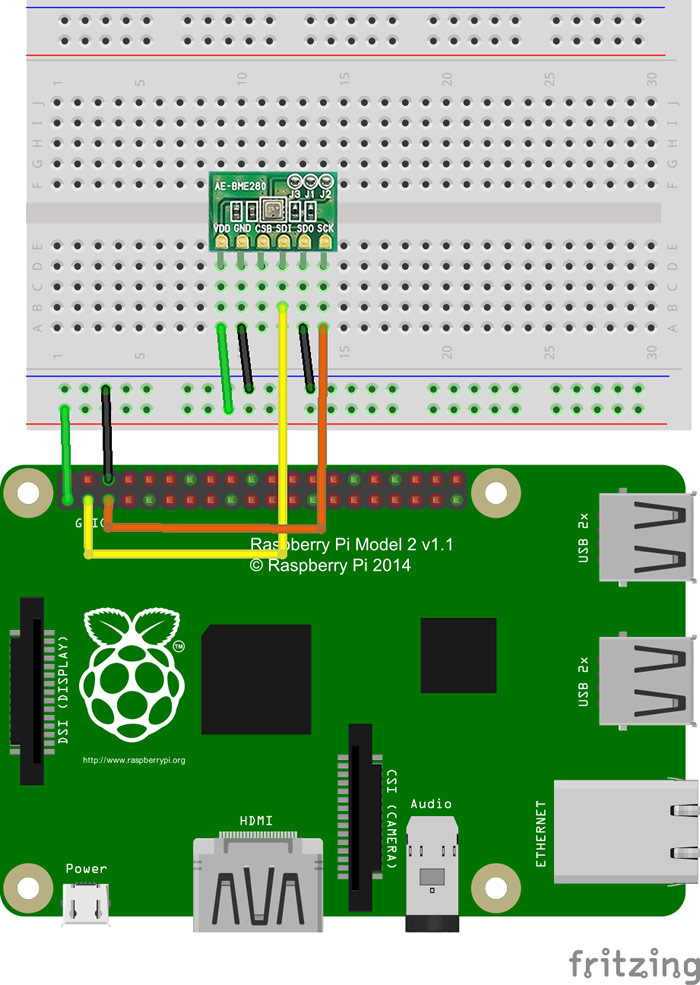## 设置 Raspberry Pi

Raspberry Pi默认禁用I2C。启用I2C的方法与启用SPI的方法相同，请参阅上一个教程：Raspberry Pi WebIOPi物联网，模拟输入编程。在菜单中选择[Preferences] – [Raspberry Pi Configuration]，然后打开“Settings ”屏幕。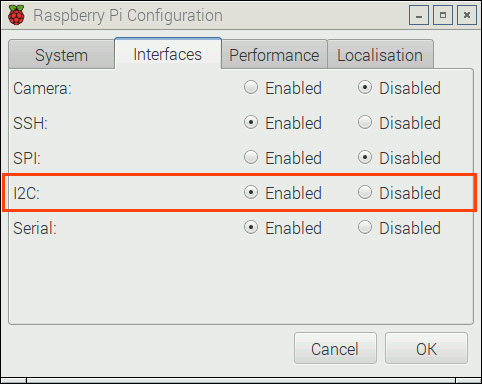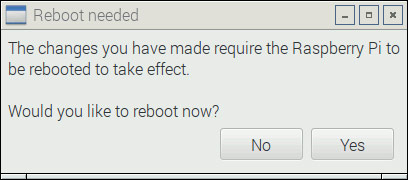（OS: 2015年11月21日发布的Raspbian Jessie版本）

sudo apt-get install i2c-tools
sudo apt-get python-smbus

sudo i2cdetect -y 1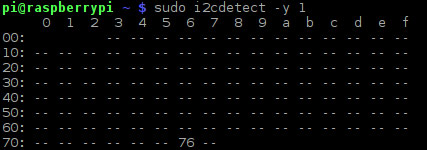76是十六进制数；代表0x76。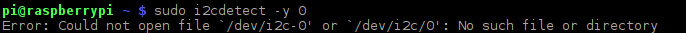sudo i2cdump -y 1 0x76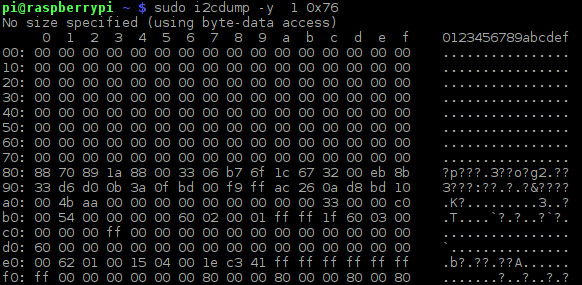SWITCHSCIENCE/BME280

python /home/pi/bme280_sample.py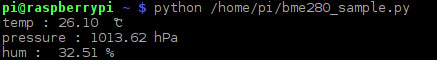/home/pi/bme280_custom.py

``````#coding: utf-8

import smbus
import time

bus_number  = 1

bus = smbus.SMBus(bus_number)

digT = []
digP = []
digH = []

t_fine = 0.0

def get_calib_param():
calib = []

for i in range (0x88,0x88+24):
for i in range (0xE1,0xE1+7):

digT.append((calib << 8) | calib)
digT.append((calib << 8) | calib)
digT.append((calib << 8) | calib)
digP.append((calib << 8) | calib)
digP.append((calib << 8) | calib)
digP.append((calib<< 8) | calib)
digP.append((calib<< 8) | calib)
digP.append((calib<< 8) | calib)
digP.append((calib<< 8) | calib)
digP.append((calib<< 8) | calib)
digP.append((calib<< 8) | calib)
digP.append((calib<< 8) | calib)
digH.append( calib )
digH.append((calib<< 8) | calib)
digH.append( calib )
digH.append((calib<< 4) | (0x0F & calib))
digH.append((calib<< 4) | ((calib >> 4) & 0x0F))
digH.append( calib )

for i in range(1,2):
if digT[i] & 0x8000:
digT[i] = (-digT[i] ^ 0xFFFF) + 1

for i in range(1,8):
if digP[i] & 0x8000:
digP[i] = (-digP[i] ^ 0xFFFF) + 1

for i in range(0,6):
if digH[i] & 0x8000:
digH[i] = (-digH[i] ^ 0xFFFF) + 1

data = []
for i in range (0xF7, 0xF7+8):
pres_raw = (data << 12) | (data << 4) | (data >> 4)
temp_raw = (data << 12) | (data << 4) | (data >> 4)
hum_raw  = (data << 8)  |  data

#compensate_T(temp_raw)
#compensate_P(pres_raw)
#compensate_H(hum_raw)
t = compensate_T(temp_raw)
p = compensate_P(pres_raw)
h = compensate_H(hum_raw)
return p + "," + t + "," + h

global  t_fine
pressure = 0.0

v1 = (t_fine / 2.0) - 64000.0
v2 = (((v1 / 4.0) * (v1 / 4.0)) / 2048) * digP
v2 = v2 + ((v1 * digP) * 2.0)
v2 = (v2 / 4.0) + (digP * 65536.0)
v1 = (((digP * (((v1 / 4.0) * (v1 / 4.0)) / 8192)) / 8)  + ((digP * v1) / 2.0)) / 262144
v1 = ((32768 + v1) * digP) / 32768

if v1 == 0:
return 0
pressure = ((1048576 - adc_P) - (v2 / 4096)) * 3125
if pressure < 0x80000000:         pressure = (pressure * 2.0) / v1     else:         pressure = (pressure / v1) * 2     v1 = (digP * (((pressure / 8.0) * (pressure / 8.0)) / 8192.0)) / 4096     v2 = ((pressure / 4.0) * digP) / 8192.0     pressure = pressure + ((v1 + v2 + digP) / 16.0)        #print "pressure : %7.2f hPa" % (pressure/100)     return "%7.2f" % (pressure/100)     def compensate_T(adc_T):     global t_fine     v1 = (adc_T / 16384.0 - digT / 1024.0) * digT     v2 = (adc_T / 131072.0 - digT / 8192.0) * (adc_T / 131072.0 - digT / 8192.0) * digT     t_fine = v1 + v2     temperature = t_fine / 5120.0     #print "temp : %-6.2f ℃" % (temperature)     return "%.2f" % (temperature)   def compensate_H(adc_H):     global t_fine     var_h = t_fine - 76800.0     if var_h != 0:         var_h = (adc_H - (digH * 64.0 + digH/16384.0 * var_h)) * (digH / 65536.0 * (1.0 + digH / 67108864.0 * var_h * (1.0 + digH / 67108864.0 * var_h)))     else:         return 0     var_h = var_h * (1.0 - digH * var_h / 524288.0)     if var_h > 100.0:
var_h = 100.0
elif var_h < 0.0:
var_h = 0.0
#print "hum : %6.2f ％" % (var_h)
return "%.2f" % (var_h)

def setup():
osrs_t = 1            #Temperature oversampling x 1
osrs_p = 1            #Pressure oversampling x 1
osrs_h = 1            #Humidity oversampling x 1
mode   = 3            #Normal mode
t_sb   = 5            #Tstandby 1000ms
filter = 0            #Filter off
spi3w_en = 0            #3-wire SPI Disable

ctrl_meas_reg = (osrs_t << 5) | (osrs_p << 2) | mode
config_reg    = (t_sb << 5) | (filter << 2) | spi3w_en
ctrl_hum_reg  = osrs_h

writeReg(0xF2,ctrl_hum_reg)
writeReg(0xF4,ctrl_meas_reg)
writeReg(0xF5,config_reg)

setup()
get_calib_param()

if __name__ == '__main__':
try:
except KeyboardInterrupt:
pass
``````

/home/pi/bme280.py

``````#coding: utf-8

import bme280_custom

import datetime

import os

dir_path = '/home/pi/bme280-data'

now = datetime.datetime.now()

filename = now.strftime('%Y%m%d')

label = now.strftime('%H:%M')

if not os.path.exists('/home/pi/bme280-data'):

os.makedirs('/home/pi/bme280-data')

f = open('/home/pi/bme280-data/'+filename+'.csv','a')

f.write("'"+label+"',"+csv+"\n")

f.close()
``````

sudo crontab -e

0-59/10 * * * * /home/pi/bme280.py

/var/www/html/bme280.php

``````<?php

\$today  = date("Ymd");

\$csv_dir  = '/home/pi/bme280-data/';

\$csv_file = \$today.'.csv';

\$grapgh   = '';

if ((\$handle = fopen(\$csv_dir.\$csv_file, "r")) !== false) {

while ((\$line = fgets(\$handle)) !== false) {

\$grapgh .= '['.rtrim(\$line).'],'.PHP_EOL;

}

fclose(\$handle);

}else{

echo 'no data';

}

?>

<html>

<script type="text/javascript">

function drawTable() {

<?php echo \$grapgh; ?>

]);

table.draw(data, {showRowNumber: true});

}

</script>

<body>

<div id="table_div"></div>

</body>

</html>
``````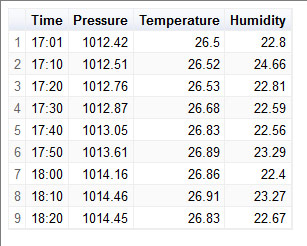Raspbian最新版本预装了一个名为“LibreOffice”的办公套件。如果您只是想查看数据，那么可以使用“LibreOffice Calc”（双击CSV文件），然后您会看到如下内容：### 相关文章

1.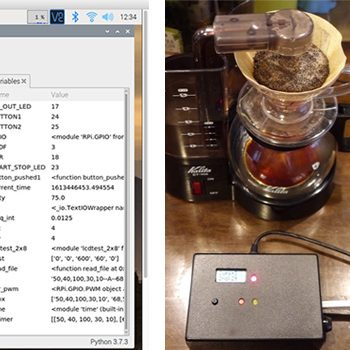#### 使用Raspberry Pi制作咖啡机控制装置 第3部分：使用Python完成控制软件

2.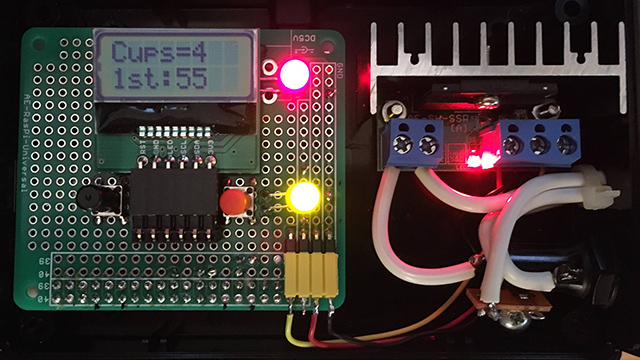#### 使用Raspberry Pi制作咖啡机控制装置 第2部分：硬件制作

3.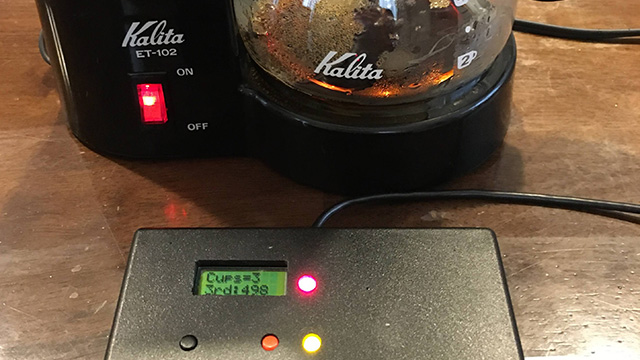#### 使用Raspberry Pi制作咖啡机控制装置 第1部分：装置概况和咖啡萃取

4.#### 数字晶体管是削减元器件、创建简单电路的第一步

5.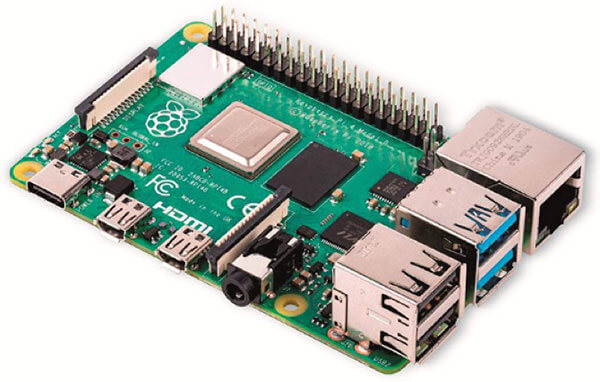#### 从历史到使用方法的全面了解！电子作品创作不可或缺的“Raspberry Pi（树莓派）”究竟是何方神…

6.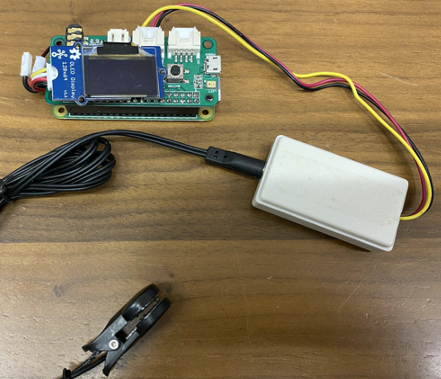#### 一款用Raspberry Pi和传感器制作的可穿戴式小型健康管理设备！第四部分•剧终篇

7.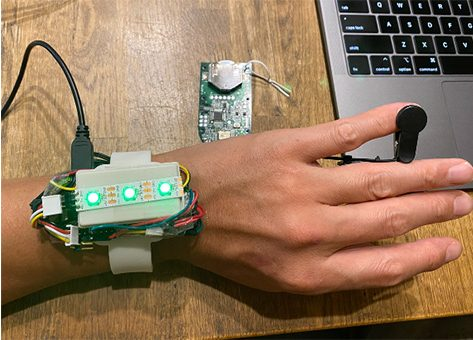#### 一款用Raspberry Pi和传感器制作的可穿戴式小型健康管理设备！第三部分

8.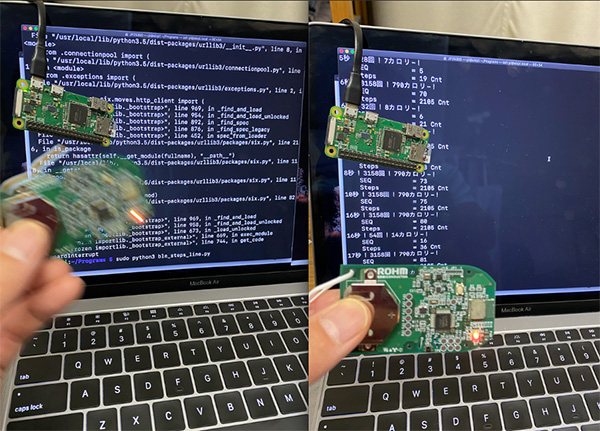#### 一款用Raspberry Pi和传感器制作的可穿戴式小型健康管理设备！第二部分

9.